# What will the initial rate be if [A] is halved and [B] is tripled? answer must be in M/s

The reaction
A+ B yields C+D
rate = k[A][B]^2
has an intial rate of 0.0220 M/s
what will the initial rate be if [A] is halved and [B] is tripled? answer must be in M/s
What will the intial rate be if [A] is tripled and [B] is halved? answer must be in M/s

Concepts and reason
The concept used to solve this problem is based on rate law of a chemical reaction.
Rate law is an equation that establishes a link between the concentration of the reactants and the rate of the reaction.

Fundamentals
For a chemical reaction,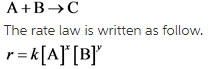Here,[A] is the concentration of reactant A,[B] is the concentration of reactant B,k is the rate constant of the reaction,x and y are the reaction orders which is an experimentally determined quantity.

Part 1
The reaction is: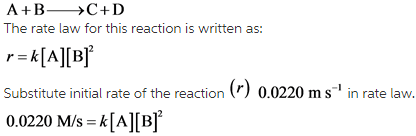An expression for initial rate is obtained using the rate law by substituting the value of initial rate.

Part 1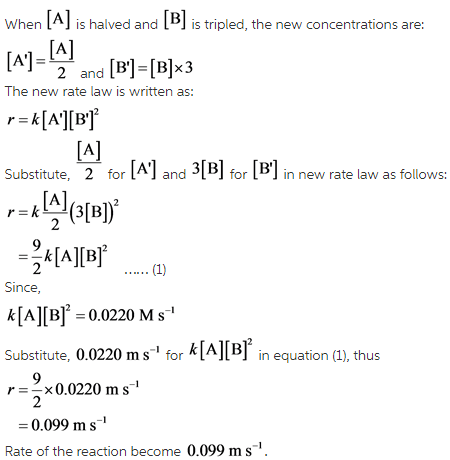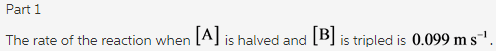Part 2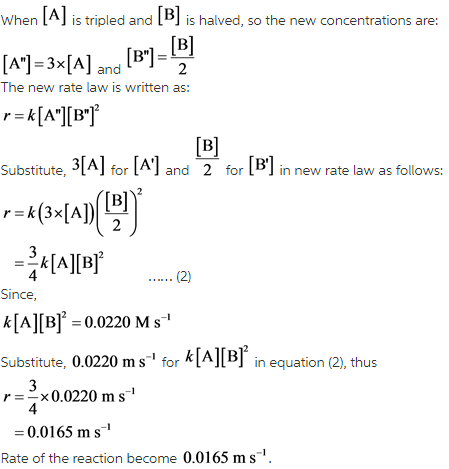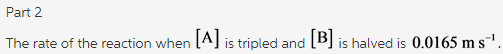When the initial concentrations of the reactants are changed by any factor, then the first step is to write the new concentrations in terms of the initial concentrations. Then, write the new rate law in terms of the initial concentrations. And then calculate the new rate law by substituting the values accordingly.